Greetings, here lies my work-log at

```
______    _      ___                _                             _____  _____  __   ______
|  ___|  | |    / _ \              | |                           / __  \|  _  |/  | |___  /
| |_ __ _| |__ / /_\ \ ___ __ _  __| | ___ _ __ ___  _   _       `' / /'| |/' |`| |    / /
|  _/ _` | '_ \|  _  |/ __/ _` |/ _` |/ _ \ '_ ` _ \| | | |        / /  |  /| | | |   / /
| || (_| | |_) | | | | (_| (_| | (_| |  __/ | | | | | |_| |      ./ /___\ |_/ /_| |_./ /
\_| \__,_|_.__/\_| |_/\___\__,_|\__,_|\___|_| |_| |_|\__, |      \_____/ \___/ \___/\_/
|_|
```

# networking and communications

## index

### /Assignment goals

• Described your design and fabrication process using words/images/screenshots
• Explained the programming process/es you used and how the microcontroller datasheet helped you
• Explained problems and how you fixed them
• Included original design files and code

### /Intro / overview

This week was the most difficult to comprehend, spent most of the allocated time researching various standards and learning about ham radios, definitely applying for a license. I've decided to learn by replicating Neil's series of bridge and node boards demonstrating 'Asynchronous bus' serial communication .

### /Designing the board and stuffing it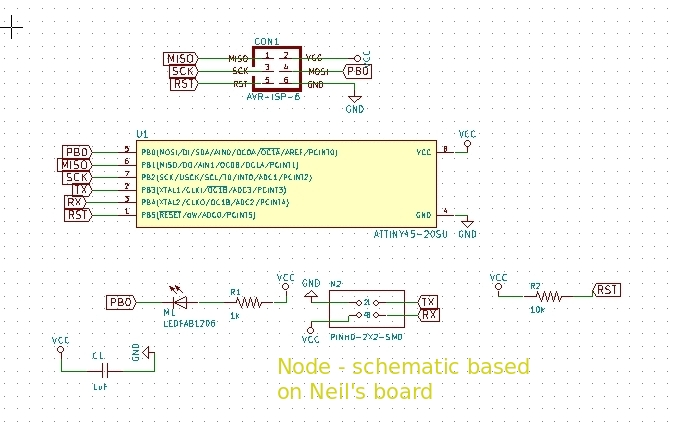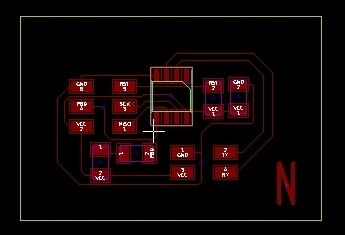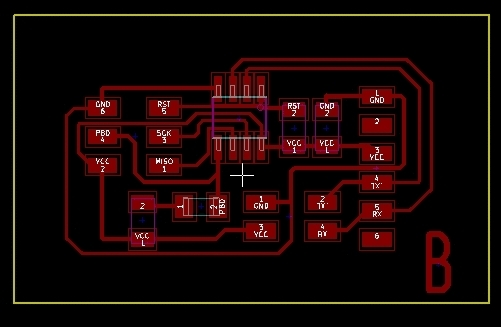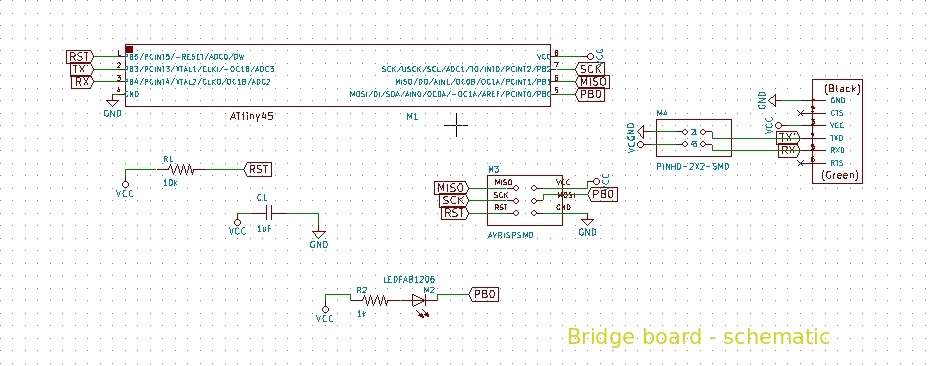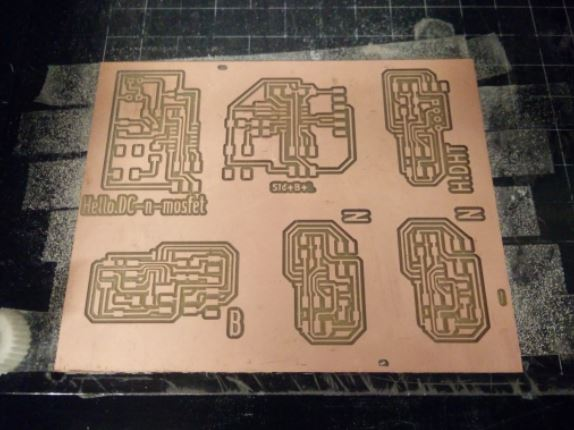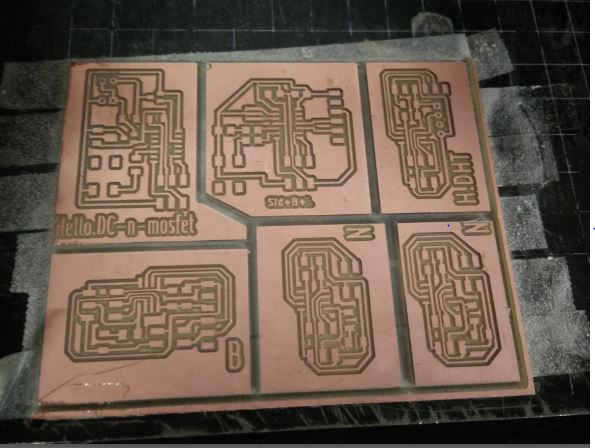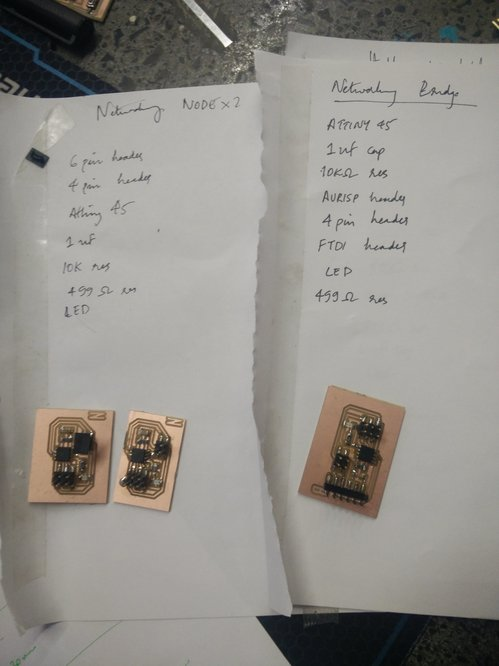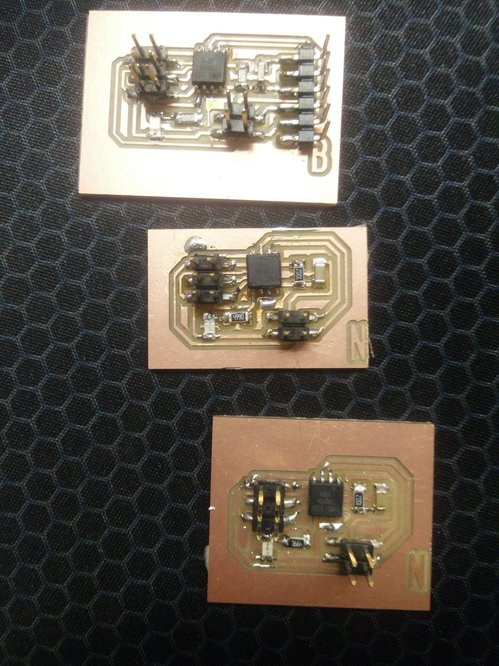### /Programming workflow

An arduino uno as ISP was used to program both the bridge and the node boards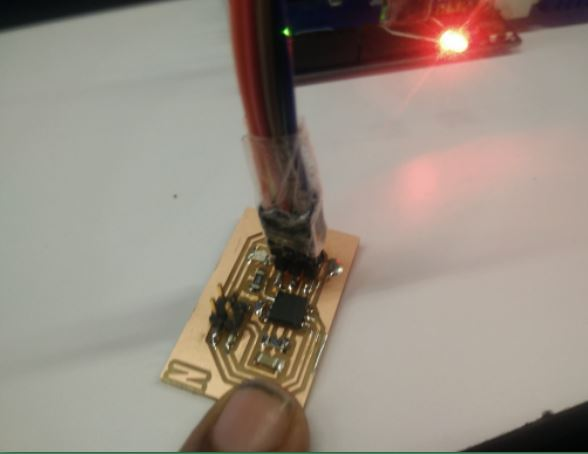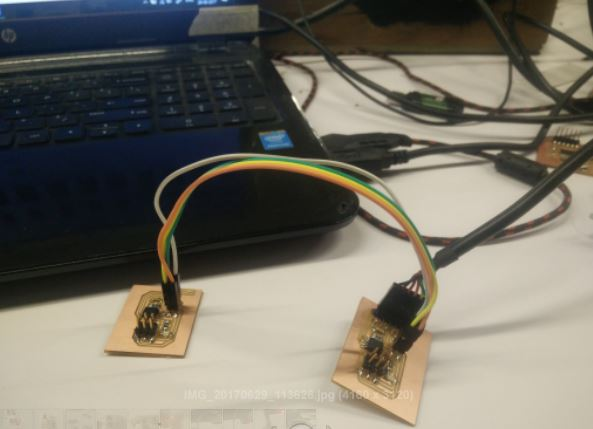### Understanding 'asynchronous bus'

• Consider the following components in the network -
• the host computer
• the bridge board and
• the node boards
• The host computer has a 'receive' and 'transmit' (TX and RX) through which it recieves and transmits messages
• So, there are two lines coming from the host computer which are called the 'bus lines' because the boards are in essence sharing these two lines for it's 'transmission' and reception' of data
• So all the local boards can listen to the host transmitting on it's transmission line without conflicts because it's just listening, but
• the crucial part in the asynchronous bus system is how the boards share the 'transmit' line to talk to the host without conflicts
• this is resolved by the following system -
• Initially, all the boards are listening and all the boards have been addressed a unique name like board no '1' or board no-'0'
• So, if the host computer wants to talk to board no -1 , the message saying that it wants to talk to board no-1 is sent to all the boards
• the board with the ID '1', momentarily takes over the line to talk to the host computer and then resumes to it's previous state which is the tri-state
• the tri-state is the concept of the pin being neither in 0 state or 1 but an output state which is seen as an input. So a pin can be in three states - 0, 1, or X 9tri-state)
• It is essential to understand that the computer sees only one line incoming, through which multiple boards take turns to talk with the host
• the bridge board is running the TX and RX pins to a header.
• the node board gets power and ground and it's TX and RX is the same 'bus' line connecting to the host
• how the code works is explained below

I used Neil's code straight up, I've begun to start learning concepts of microcontrollers like their respective registers, and different memory types whilst trying to understand the code.

Here is what i understand of the code -

• #define node_id '0' //this is where the board is given an ID
• ``````while (1) {
get_char(&serial_pins, serial_pin_in, &chr);
flash();
if (chr == node_id) {
output(serial_direction, serial_pin_out);
static const char message[] PROGMEM = "node ";
put_string(&serial_port, serial_pin_out, (PGM_P) message);
put_char(&serial_port, serial_pin_out, chr);
put_char(&serial_port, serial_pin_out, 10); // new line
led_delay();
flash();
input(serial_direction, serial_pin_out);
}
}
} ``````

this is the main loop where, the board is constantly listening for a character, and the 'if' statement "`(if (chr == node_id))`" will run if the board's 'ID' matches the one being called out by the host computer.
• If the ID matches, the serial pin becomes output through this code
``output(serial_direction, serial_pin_out);``
• then it sends out the message - static const char message[] PROGMEM = "node ";
• After that, the LED flashes
```led_delay(); flash();```
• and at last the pin becomes an input again
`input(serial_direction, serial_pin_out);`
• And hence, a master-slave relation is established; where the host computer is the master and the boards are the slaves.

Code on the local boards

``` ``` //
//
// hello.bus.45.c
//
// 9600 baud serial bus hello-world
//
// Neil Gershenfeld
// 11/24/10
//
// (c) Massachusetts Institute of Technology 2010
// Permission granted for experimental and personal use;
// license for commercial sale available from MIT.
//

#include
#include
#include
#include

#define output(directions,pin) (directions |= pin) // set port direction for output
#define input(directions,pin) (directions &= (~pin)) // set port direction for input
#define set(port,pin) (port |= pin) // set port pin
#define clear(port,pin) (port &= (~pin)) // clear port pin
#define pin_test(pins,pin) (pins & pin) // test for port pin
#define bit_test(byte,bit) (byte & (1 << bit)) // test for bit set
#define bit_delay_time 100 // bit delay for 9600 with overhead
#define bit_delay() _delay_us(bit_delay_time) // RS232 bit delay
#define half_bit_delay() _delay_us(bit_delay_time/2) // RS232 half bit delay
#define led_delay() _delay_ms(100) // LED flash delay

#define led_port PORTB
#define led_direction DDRB
#define led_pin (1 << PB0)

#define serial_port PORTB
#define serial_direction DDRB
#define serial_pins PINB
#define serial_pin_in (1 << PB3)
#define serial_pin_out (1 << PB4)

#define node_id '0'

void get_char(volatile unsigned char *pins, unsigned char pin, char *rxbyte) {
//
// read character into rxbyte on pins pin
//    assumes line driver (inverts bits)
//
*rxbyte = 0;
while (pin_test(*pins,pin))
//
// wait for start bit
//
;
//
// delay to middle of first data bit
//
half_bit_delay();
bit_delay();
//
// unrolled loop to read data bits
//
if pin_test(*pins,pin)
*rxbyte |= (1 << 0);
else
*rxbyte |= (0 << 0);
bit_delay();
if pin_test(*pins,pin)
*rxbyte |= (1 << 1);
else
*rxbyte |= (0 << 1);
bit_delay();
if pin_test(*pins,pin)
*rxbyte |= (1 << 2);
else
*rxbyte |= (0 << 2);
bit_delay();
if pin_test(*pins,pin)
*rxbyte |= (1 << 3);
else
*rxbyte |= (0 << 3);
bit_delay();
if pin_test(*pins,pin)
*rxbyte |= (1 << 4);
else
*rxbyte |= (0 << 4);
bit_delay();
if pin_test(*pins,pin)
*rxbyte |= (1 << 5);
else
*rxbyte |= (0 << 5);
bit_delay();
if pin_test(*pins,pin)
*rxbyte |= (1 << 6);
else
*rxbyte |= (0 << 6);
bit_delay();
if pin_test(*pins,pin)
*rxbyte |= (1 << 7);
else
*rxbyte |= (0 << 7);
//
// wait for stop bit
//
bit_delay();
half_bit_delay();
}

void put_char(volatile unsigned char *port, unsigned char pin, char txchar) {
//
// send character in txchar on port pin
//    assumes line driver (inverts bits)
//
// start bit
//
clear(*port,pin);
bit_delay();
//
// unrolled loop to write data bits
//
if bit_test(txchar,0)
set(*port,pin);
else
clear(*port,pin);
bit_delay();
if bit_test(txchar,1)
set(*port,pin);
else
clear(*port,pin);
bit_delay();
if bit_test(txchar,2)
set(*port,pin);
else
clear(*port,pin);
bit_delay();
if bit_test(txchar,3)
set(*port,pin);
else
clear(*port,pin);
bit_delay();
if bit_test(txchar,4)
set(*port,pin);
else
clear(*port,pin);
bit_delay();
if bit_test(txchar,5)
set(*port,pin);
else
clear(*port,pin);
bit_delay();
if bit_test(txchar,6)
set(*port,pin);
else
clear(*port,pin);
bit_delay();
if bit_test(txchar,7)
set(*port,pin);
else
clear(*port,pin);
bit_delay();
//
// stop bit
//
set(*port,pin);
bit_delay();
//
// char delay
//
bit_delay();
}

void put_string(volatile unsigned char *port, unsigned char pin, PGM_P str) {
//
// send character in txchar on port pin
//    assumes line driver (inverts bits)
//
static char chr;
static int index;
index = 0;
do {
put_char(&serial_port, serial_pin_out, chr);
++index;
} while (chr != 0);
}

void flash() {
//
// LED flash delay
//
clear(led_port, led_pin);
led_delay();
set(led_port, led_pin);
}

int main(void) {
//
// main
//
static char chr;
//
// set clock divider to /1
//
CLKPR = (1 << CLKPCE);
CLKPR = (0 << CLKPS3) | (0 << CLKPS2) | (0 << CLKPS1) | (0 << CLKPS0);
//
// initialize output pins
//
set(serial_port, serial_pin_out);
input(serial_direction, serial_pin_out);
set(led_port, led_pin);
output(led_direction, led_pin);
//
// main loop
//
while (1) {
get_char(&serial_pins, serial_pin_in, &chr);
flash();
if (chr == node_id) {
output(serial_direction, serial_pin_out);
static const char message[] PROGMEM = "node ";
put_string(&serial_port, serial_pin_out, (PGM_P) message);
put_char(&serial_port, serial_pin_out, chr);
put_char(&serial_port, serial_pin_out, 10); // new line
led_delay();
flash();
input(serial_direction, serial_pin_out);
}
}
}   ``````
` `